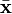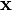# Barycentre

In geometry, the barycentre or centre of mass or centre of gravity of a system of particles or a rigid body is a point at which various systems of force may be deemed to act. The gravitational attraction of a mass is centred at its barycentre (hence the term "centre of gravity"), and the (classical) angular momentum of the mass resolves into components related to the rotation of the body about its barycentre and the angular movement of the barycentre.

The barycentre is located as an "average" of the masses involved. For a system of n point particles of mass mi located at position vectors$\mathbf{x}_i$, the barycentre$\bar{\mathbf{x}}$ is defined by$\left( \sum_{i=1}^n m_i \right) \bar{\mathbf{x}} = \sum_{i=1}^n m_i \mathbf{x}_i . \,$

For a solid body B with mass density$\rho(\mathbf{x})$ at position$\mathbf{x}$, with total mass$M = \iiint_B \rho(\mathbf{x}) \mathrm{d}\mathbf{x} , \,$

the barycentre is given by$M \bar{\mathbf{x}} = \iiint_B \mathbf{x} \rho(\mathbf{x}) \mathrm{d}\mathbf{x} . \,$